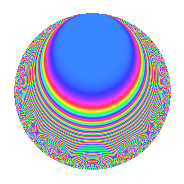# Properties

 Label 231.2.gLevel 231 Weight 2 Character orbit g Rep. character $$\chi_{231}(197,\cdot)$$ Character field $$\Q$$ Dimension 24 Newform subspaces 1 Sturm bound 64 Trace bound 0

# Related objects

## Defining parameters

 Level: $$N$$ = $$231 = 3 \cdot 7 \cdot 11$$ Weight: $$k$$ = $$2$$ Character orbit: $$[\chi]$$ = 231.g (of order $$2$$ and degree $$1$$) Character conductor: $$\operatorname{cond}(\chi)$$ = $$33$$ Character field: $$\Q$$ Newform subspaces: $$1$$ Sturm bound: $$64$$ Trace bound: $$0$$

## Dimensions

The following table gives the dimensions of various subspaces of $$M_{2}(231, [\chi])$$.

Total New Old
Modular forms 36 24 12
Cusp forms 28 24 4
Eisenstein series 8 0 8

## Trace form

 $$24q - 6q^{3} + 24q^{4} + 10q^{9} + O(q^{10})$$ $$24q - 6q^{3} + 24q^{4} + 10q^{9} - 20q^{12} - 10q^{15} - 8q^{16} - 12q^{25} - 20q^{31} + 14q^{33} - 8q^{34} - 12q^{36} + 4q^{37} + 6q^{45} - 48q^{48} - 24q^{49} - 28q^{55} + 44q^{58} + 32q^{60} - 52q^{64} + 12q^{66} - 4q^{67} + 54q^{69} - 20q^{70} + 68q^{75} - 20q^{78} + 2q^{81} + 16q^{82} - 44q^{88} + 24q^{91} + 26q^{93} - 12q^{97} - 6q^{99} + O(q^{100})$$

## Decomposition of $$S_{2}^{\mathrm{new}}(231, [\chi])$$ into newform subspaces

Label Dim. $$A$$ Field CM Traces $q$-expansion
$$a_2$$ $$a_3$$ $$a_5$$ $$a_7$$
231.2.g.a $$24$$ $$1.845$$ None $$0$$ $$-6$$ $$0$$ $$0$$

## Decomposition of $$S_{2}^{\mathrm{old}}(231, [\chi])$$ into lower level spaces

$$S_{2}^{\mathrm{old}}(231, [\chi]) \cong$$ $$S_{2}^{\mathrm{new}}(33, [\chi])$$$$^{\oplus 2}$$

## Hecke Characteristic Polynomials

There are no characteristic polynomials of Hecke operators in the database